#### Multiple, independent double integrators

Now consider generalizing to a vector ofdouble integrators. In this case,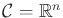and each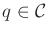is an-dimensional vector. There areaction variables anddouble integrators of the form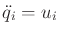. The action space for each variable is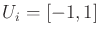(once again, any acceleration bound can be used). The phase spaceis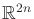, and each point is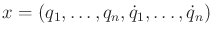. Theth double integrator produces two scalar equations of the phase transition equation: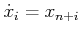and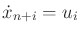.

Even though there aredouble integrators, they are decoupled in the state transition equation. The phase of one integrator does not depend on the phase of another. Therefore, the ideas expressed so far can be extended in a straightforward way to obtain a lattice over. Each action is an-dimensional vector. Eachis discretized to yield values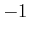, 0, and. There are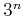edges emanating from any lattice point for which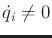for all. For any double integrator for which, there are only two choices because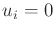produces no motion. The projection of the reachability graph down to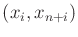for anyfromtolooks exactly like Figure 14.13 and characterizes the behavior of theth integrator.

The standard search algorithms can be applied to the lattice over. Breadth-first search once again yields solutions that are approximately time-optimal. Resolution completeness can be obtained again by boundingand allowing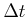to converge to zero. Now that there are more dimensions, a complicated obstacle region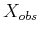can be removed from. The traversal of each edge then requires collision detection along each edge of the graph. Note that the state trajectories are linear or parabolic arcs. Numerical integration is not needed because (14.22) already gives the closed-form expression for the state trajectory.

Steven M LaValle 2020-08-14